# Logistic Regression in Python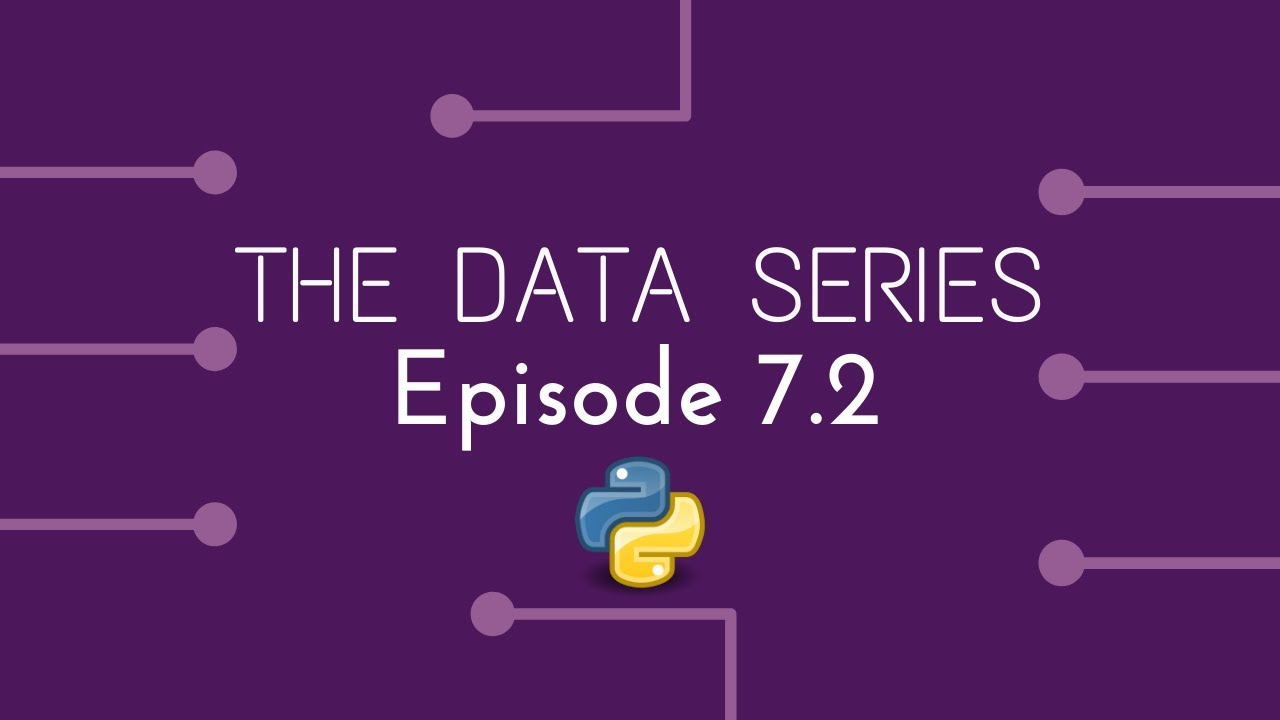Logistic Regression in Python. Logistic Regression is a Machine Learning classification algorithm that is used to predict the probability of a categorical dependent variable. Build your knowledge in Data Science from complete beginner to expert. You will competent in all fields of Data science and will have the ability to build top tier data science models which will be useful for personal projects and employment.

You can view and use the code and data used in this episode here: Link

## Objective

Predict weather it will rain tomorrow in Albury, Australia given the following data: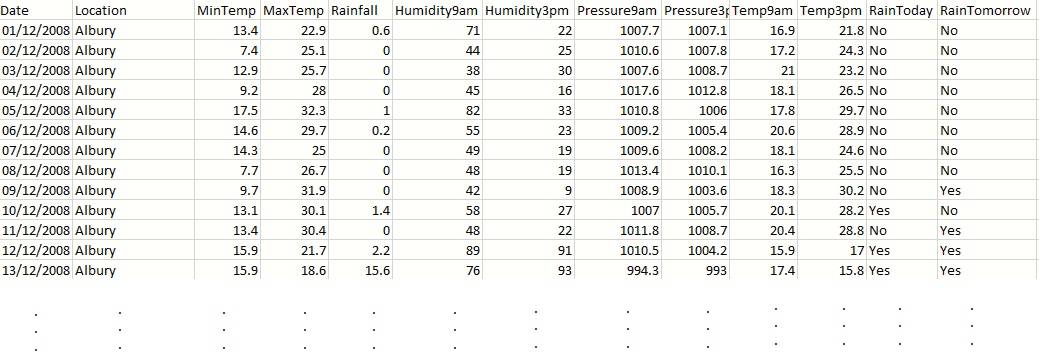• We store our data in the variable df short for data frame.
• df.shape gives the number of rows and columns in our data.
• df.head displays the first few rows of data on our notebook.
``````## Read the dataimport pandas as pddf = pd.read_csv("D:\ProjectData\weatherAlbury.csv")
print('Size of weather data frame is :',df.shape)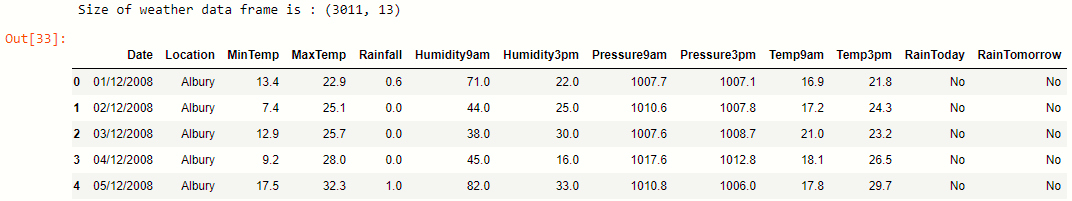We see that our weather data has 3011 rows and 13 columns.

## Pre-processing our data

### Removing missing entries and converting to binary

In this episode, for the pre-processing, we will just be removing any rows which contain a NA. (Not Applicable). This is commonly done if we want to apply a model on our data quickly.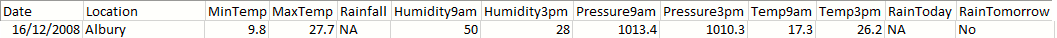Since we are removing a whole row — we may be loosing valuable data. For example we are removing the row shown above, even though we still have data for MinTemp, MaxTemp, Humidity and more.

Often we replace NA with the mean or mode of that column — we will discuss this in a future episode.

We also replace all yes and no’s with binary numbers 0 and 1, since models work with numbers not words.

``````## Preprocess the datadf = df.dropna()
print("new shape:" ,df.shape)## Replace yes and no with 1 and 0df['RainToday'].replace({'No': 0, 'Yes': 1},inplace = True)
df['RainTomorrow'].replace({'No': 0, 'Yes': 1},inplace = True)``````Previously we had 3011 rows and now 2981 — so we have removed 30 rows of data.

### Defining our Model’s Features

We define our model’s features using the following code, in this case we are using all of our features to predict weather it will rain tomorrow in Albury.

``````X = df[["MinTemp", "MaxTemp", "Rainfall", "Humidity9am", "Humidity3pm", "Pressure9am", "Pressure3pm", "Temp9am", "Temp3pm", "RainToday"]]
y = df.RainTomorrow``````

### Splitting our data

We split our data into training and test data in order to cross-validate our model.

Here we will be using 80% training data and 20% test data. To split our data we must import train_test_split from the sklearn library. We also randomly-shuffle our data to prevent any bias problems. random_state = 42, keeps the shuffling algorithm the same, so we produce consistent results which is easier to evaluate.

``X_train,X_test,y_train,y_test = train_test_split(X,y,test_size=0.20, random_state=42)``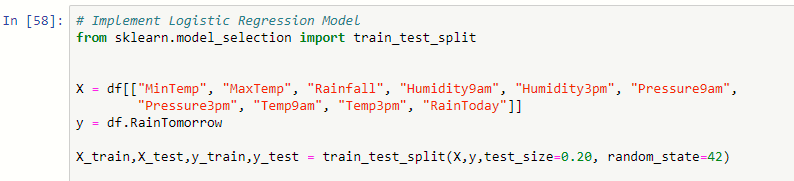## Building our Logistic Regression Model

We import the logistic regression function from the sci-kit learn library and apply it to our data.

We use y_pred to get a set of predicted values from our test data, in order to evaluate our model.

``````logreg = LogisticRegression()
logreg.fit(X_train,y_train)y_pred = logreg.predict(X_test)``````

Putting our code together we get:

``````## Implement Logistic Regression Model
from sklearn.model_selection import train_test_splitX = df[["MinTemp", "MaxTemp", "Rainfall", "Humidity9am", "Humidity3pm", "Pressure9am",
"Pressure3pm", "Temp9am", "Temp3pm", "RainToday"]]
y = df.RainTomorrowX_train,X_test,y_train,y_test = train_test_split(X,y,test_size=0.20, random_state=42)logreg = LogisticRegression()
logreg.fit(X_train,y_train)y_pred = logreg.predict(X_test)``````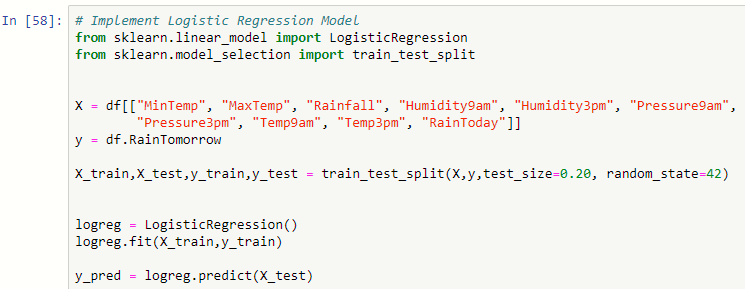## Data Science Projects | Data Science | Machine Learning | Python

Practice your skills in Data Science with Python, by learning and then trying all these hands-on, interactive projects, that I have posted for you.

## Data Science Projects | Data Science | Machine Learning | Python

Practice your skills in Data Science with Python, by learning and then trying all these hands-on, interactive projects, that I have posted for you.

## Data Science Projects | Data Science | Machine Learning | Python

Practice your skills in Data Science with Python, by learning and then trying all these hands-on, interactive projects, that I have posted for you.

## Data Science Projects | Data Science | Machine Learning | Python

Practice your skills in Data Science with Python, by learning and then trying all these hands-on, interactive projects, that I have posted for you.

## Data Science Projects | Data Science | Machine Learning | Python

Practice your skills in Data Science with Python, by learning and then trying all these hands-on, interactive projects, that I have posted for you.